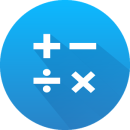Math: mental math games, multiplication table

2.9.5
4.5 / 4 reviews

A trainer app for improving math skills. Access a series of training exercises for memorizing and practicing basic and advanced mathematical equations for quick calculations with no supporting software. The suite contains multiple types of math games organized according to relevant methods.

App info

500 000+
Size:
9.07 MB
Updated:
2.9.5Math: mental math games, multiplication table 2.9.5

Previous versions

Math: mental math games, multiplication table
9.03 MB
Math: mental math games, multiplication table
9.05 MB
Math: mental math games, multiplication table
8.97 MB
Math: mental math games, multiplication table
9.05 MB
Math: mental math games, multiplication table
8.92 MB
Math: mental math games, multiplication table
8.92 MB
Math: mental math games, multiplication table
8.00 MB
Math: mental math games, multiplication table
9.70 MB
Math: mental math games, multiplication table
9.30 MB
Math: mental math games, multiplication table
9.41 MB

AlternativesTons of fun math learning games to suit all children ages 3 – 8.FreeAn app for improving singing skills.FreeDebugging app that can also hack into games for cheating purposes.FreeA collection of 12 games designed to be educational for children.FreeEducational game that helps build the vocabulary.Free